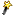关于编辑器KindEditor自动过滤首行缩进和全角空格的解决方法

KindEditor 4.1.11：
kindeditor-all.js 文件

var re = /(\s*)<(\/)?([\w\-:]+)((?:\s+|(?:\s+[\w\-:]+)|(?:\s+[\w\-:]+=[^\s"'<>]+)|(?:\s+[\w\-:"]+="[^"]*")|(?:\s+[\w\-:"]+='[^']*'))*)(\/)?>(\s*)/g;